# Understanding that QCD is not CP invariant

• A
JD_PM
TL;DR Summary
I want to understand how to show that the gauge-invariant, renormalizable term ##\frac 1 2 G_i^{\mu \nu} \varepsilon_{\mu \nu \rho \sigma} G^{\rho \sigma}_i## breaks the CP symmetry of QCD
In "CP violation" book by Bigi and Sanda (section 8.2.1. QCD), I read that "the QCD Lagrangian is invariant under CP transformations" and wanted to prove it.

The QCD Lagrangian is given by

\begin{equation}
\mathscr{L}_{QCD} = \bar \Psi^f [i \gamma^{\mu}D_{\mu} -m_f] \Psi^f - \frac 1 4 G_{i \mu \nu}G_i^{\mu \nu} \tag{1}
\end{equation}

Where ##f## stands for quark flavor and

\begin{equation*}
\Psi^f = \begin{pmatrix}
\psi_r^f \\
\psi_g^f \\
\psi_b^f \\
\end{pmatrix}, \quad G_i^{\mu \nu} := F_i^{\mu \nu} + g_s f_{ijk} A_j^{\mu} A_k^{\nu}, \quad F_i^{\mu \nu} := \partial^{\nu} A^{\mu}_i - \partial^{\mu} A^{\nu}_i
\end{equation*}

Where ##f_{ijk}## is the so-called structure constant, which is totally antisymmetric.

The covariant derivative is given by

\begin{equation*}
D_{\mu} \Psi^f = \left[ \partial_{\mu} + ig_s \lambda_j A_j^{\mu}/2 \right] \Psi^f
\end{equation*}

Where ##\lambda## are the Gell-Mann matrices.

In class we were taught CP using a particular representation of the gamma matrices: the Weyl representation (alternative form). So let us use the same here. It follows that

\begin{equation*}
\psi_{cp} = i \gamma_2 \psi^*, \quad \bar \Psi \chi \to \bar \Psi_{cp} \chi_{cp} = \bar \chi \Psi, \quad \bar \Psi \gamma_{\mu} \chi \to \bar \Psi_{cp} \gamma_{\mu} \chi_{cp} = -\bar \chi \gamma_{\mu} \Psi, \quad A^{\mu}_{cp} = -A^{\mu}
\end{equation*}

Using the above transformations I see that ##(1)## is CP invariant (please let me know if you want me to give the proof).

Then Bigi and Sanda asserted that QCD is actually not CP invariant, because there exists a gauge-invariant and renormalizable operator that can be added to ##(1)##

\begin{equation}
\frac 1 2 G_i^{\mu \nu} \varepsilon_{\mu \nu \rho \sigma} G^{\rho \sigma}_i \tag{2}
\end{equation}

However, I do not see why the presence of the totally antisymmetric tensor ##\varepsilon_{\mu \nu \rho \sigma}## breaks CP. I get that it is CP invariant as well, just as the ##\frac 1 4 G_{i \mu \nu}G_i^{\mu \nu}## term... What am I missing?

Thank you!•arivero

Staff Emeritus
Homework Helper
Gold Member
The Levi-Civita symbol represents the components of a pseudo-tensor, not a tensor.

•vanhees71 and JD_PM
JD_PM
Hi Orodruin! It is nice to talk to you again! You were extremely patient with me in the past (I recall the extensive discussion in the Adv. HW Forum we had) and I appreciate itI see, but how to construct a rule to show we pick a -ive sign when CP transformations are applied to a pseudo-tensor?

All I know is the set of rules regarding scalar ##S## , pseudoscalar ##P##, vector ##V_{\mu}## and axial vector ##A_{\mu}## fields i.e.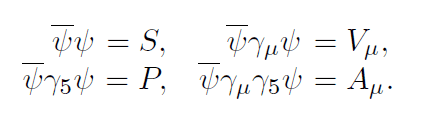Staff Emeritus
Homework Helper
Gold Member
In this case you have a pseudo-tensor fully contracted with indices from tensors. The result of this is a pseudo-scalar, which therefore picks up a - sign under parity transformations.

•vanhees71, dextercioby, arivero and 1 other person
Staff Emeritus
I don't understand how this answer explains the quote from the original post:

QCD is actually not CP invariant, because there exists a gauge-invariant and renormalizable operator that can be added to (1)

How can the possibility of adding an additional term to the lagrangian affect whether QCD is CP invariant? Is the pseudo-scalar term present in the QCD lagrangian, or not? If not, then how can that term matter to whether QCD is CP invariant?

Or maybe it's just that we can't rule out the possibility of an as-yet-unobserved CP-violating term?

•Paul Colby
Staff Emeritus
Homework Helper
Gold Member
I don't understand how this answer explains the quote from the original post:

How can the possibility of adding an additional term to the lagrangian affect whether QCD is CP invariant? Is the pseudo-scalar term present in the QCD lagrangian, or not? If not, then how can that term matter to whether QCD is CP invariant?

Or maybe it's just that we can't rule out the possibility of an as-yet-unobserved CP-violating term?
This is known as the strong CP-problem. There is no symmetry a priori forbidding the term and therefore the question is why it is unobservably small (or even zero). In essence it is a question of the alignment of the multiplier of this term and the chiral quark mass phase. This therefore becomes a fine-tuning problem. Attempts to resolve this result in things like the QCD axion, which would explain why CP is restored dynamically.

The Wikipedia page on the strong CP-problem is rather short, but offers a brief overview.

•arivero and vanhees71
Staff Emeritus
Equation (2) is a little misleading because of the 1/2. It makes it look like its magnitude is determined. It's not, but the indeterminancy is rolled into the coupling constant. If you instead pulled it out in front and replaced the 1/2 by ε (or if you prefer ε/2) the Strong CP Problem asks the question why is ε (as far as we can tell) identically zero?

The general thought is that some new symmetry requires it. The most commonly invoked one is something called Peccei-Quinn, and you can read more about it here: https://arxiv.org/abs/hep-ph/9506229

•JD_PM, vanhees71, arivero and 1 other person
JD_PM
Thank you all for the helpful replies.

While having a closer look at the Lagrangian ##(1)## I noticed I made a mistake when proving Charge-conjugation invariance in the term ##\propto F_{\mu \nu} A^{\mu} A^{\nu}##. Imposing the rule ##A_c^{\mu} = - A^{\mu}## I get that such term is not C invariant i.e.

\begin{align*}
(F_{\mu \nu})_c A^{\mu}_c A^{\nu}_c &= (\partial_{\nu} A_{\mu} - \partial_{\mu} A_{\nu})_c A^{\mu}_c A^{\nu}_c \\
&= (\partial_{\nu} A_{\mu})_c A^{\mu}_c A^{\nu}_c - (\partial_{\mu} A_{\nu})_c A^{\mu}_c A^{\nu}_c \\
&= -F_{\mu \nu} A^{\mu} A^{\nu}
\end{align*}

But I do not see the error I am making...

JD_PM
Alright, I have compared how Bigi & Sanda show that the term ##G \cdot G := \frac 1 4 G_{i \mu \nu}G_i^{\mu \nu}## is CP invariant and that the term ##G \cdot \tilde G := \varepsilon_{\mu \nu \rho \sigma} G_i^{\mu \nu}G_i^{\rho \sigma}## is not to how I have been doing it so far.

They proceed in a different way, based on "the colour electric and magnetic fields ##\vec E_i## and ##\vec B_i##". They state that

\begin{equation}
G \cdot G \propto \sum_a |\vec E_a|^2 + \sum_a |\vec B_a|^2 \tag{3}
\end{equation}

\begin{equation}
G \cdot \tilde G \propto \sum_a \vec E_a \cdot \vec B_a \tag{4}
\end{equation}

I understand that the RHS of ##(3)## and ##(4)## is parity invariant (given that ##(\vec E_i)_p = -\vec E_i## and ##(\vec B_i)_p = \vec B_i##) but I do not understand why ##(3)## and ##(4)## hold.

Here's the section of the book I am talking about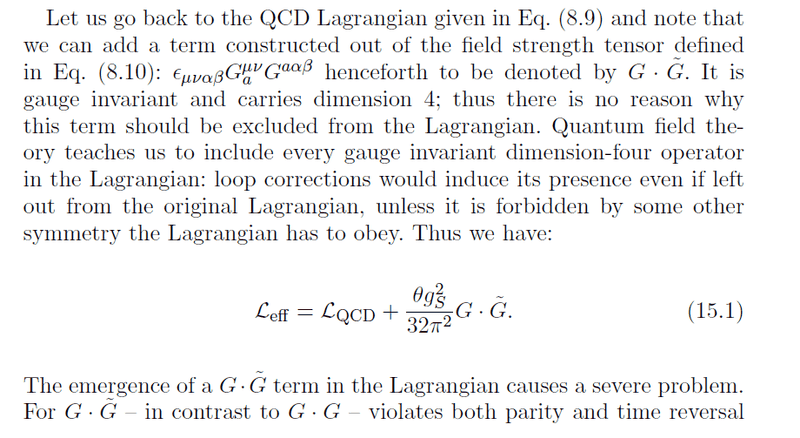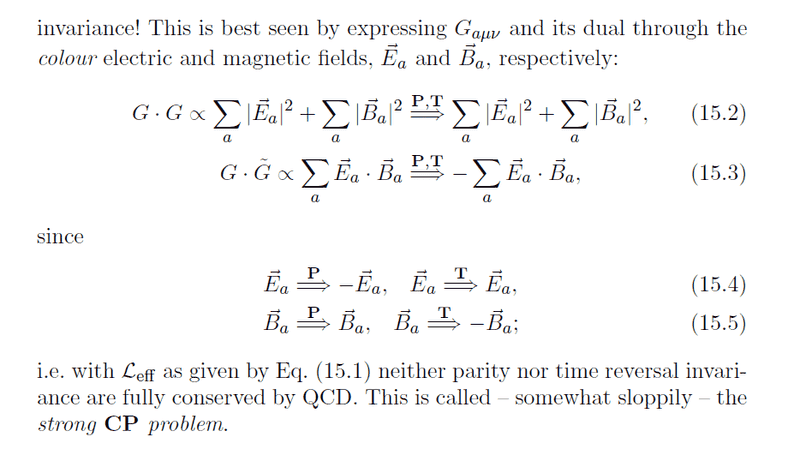PS: hello @Gaussian97 I wanted to ping you, as you might be interested in the topic.

Homework Helper
Hi @JD_PM, two things to comment on here.
First of all regarding your last question:
\begin{equation}
G \cdot G \propto \sum_a |\vec E_a|^2 + \sum_a |\vec B_a|^2 \tag{3}
\end{equation}

\begin{equation}
G \cdot \tilde G \propto \sum_a \vec E_a \cdot \vec B_a \tag{4}
\end{equation}

I understand that the RHS of ##(3)## and ##(4)## is parity invariant (given that ##(\vec E_i)_p = -\vec E_i## and ##(\vec B_i)_p = \vec B_i##) but I do not understand why ##(3)## and ##(4)## hold.
Those relations are very common in electrodynamics, I've never worked with them in QCD. In QED they follow very straightforward from the definitions
$$E^i = F^{i0}, \qquad B^i = -\frac{1}{2}\varepsilon^{ijk}F_{jk}$$

So I would try to prove those inequalities starting from
$$E_a^i = G_a^{i0}, \qquad B_a^i = -\frac{1}{2}\varepsilon^{ijk}G^a_{jk}$$
But keep in mind that since its a non-abelian theory these definitions could be slightly different...

In any case, I think is easier to prove these properties starting from the fields themselves.
\begin{equation*}
\psi_{cp} = i \gamma_2 \psi^*, \quad \bar \Psi \chi \to \bar \Psi_{cp} \chi_{cp} = \bar \chi \Psi, \quad \bar \Psi \gamma_{\mu} \chi \to \bar \Psi_{cp} \gamma_{\mu} \chi_{cp} = -\bar \chi \gamma_{\mu} \Psi, \quad A^{\mu}_{cp} = -A^{\mu}
\end{equation*}
I don't know where do you get those relations, but I don't agree with how ##A^\mu## transform.
Since ##A^\mu## is a vector quantity it transforms under P in the same way as any vector, the spatial components change sign and the temporal is left invariant (there may be an extra phase, but we don't care about it now). So
$$G_{a\text{P}}^\mu = \mathscr{P}^\mu{}_\nu G_{a}^\nu$$
where
$$\mathscr{P}^\mu{}_\nu = \begin{pmatrix}1 & 0 & 0 & 0 \\ 0 & -1 & 0 & 0 \\ 0 & 0 & -1 & 0 \\ 0 & 0 & 0 & -1\end{pmatrix}$$

Knowing that ##\partial_\mu## is also a vector quantity, is almost trivial to prove that ##G_{a\mu\nu}G^{\mu\nu}_a## is P invariant but ##\tilde{G}_{a\mu\nu}G^{\mu\nu}_a## is not.

Since you are dealing with real fields, the transformation under C conjugation is trivial and doesn't affect the result.

•JD_PM
JD_PM

So I would try to prove those inequalities starting from
$$E_a^i = G_a^{i0}, \qquad B_a^i = -\frac{1}{2}\varepsilon^{ijk}G^a_{jk}$$
But keep in mind that since its a non-abelian theory these definitions could be slightly different...

Alright, so let us assume the equations you provided. Let us drop the colour index ##a## for simplicity. Working with the ##(+---)## signature

\begin{align*}
G\cdot G &= \frac 1 4 G_{i\mu \nu}G^{\mu \nu}_i \\
&= \frac 1 4 \left( G_{i00}G^{00}_i - G_{ijk}G^{jk}_i\right) \\
&= \frac 1 4 \left( E_i^2 - ...\right)
\end{align*}

Mmm but I do not see how to evaluate the ##G_{ijk}G^{jk}_i## object given ##B_a^i = -\frac{1}{2}\varepsilon^{ijk}G^a_{jk}##. Besides I think ## G_{i00}G^{00}_i = E_i^2## is wrong, given that in the definition you provided we have an spatial index ##i##, while I only have time indices...

I don't know where do you get those relations, but I don't agree with how ##A^\mu## transform.

To start off, we make the following choice: working with a particular representation of the gamma matrices; the Weyl representation (alternative form) i.e.

\begin{equation*}
\gamma^0 = \begin{pmatrix}
0 & -\Bbb{1} \\
-\Bbb{1} & 0 \\
0 & \sigma_j \\
-\sigma_j & 0 \\
\end{pmatrix}
\end{equation*}

\begin{equation*}
\gamma_5 := i\gamma^0\gamma^1\gamma^2\gamma^3 = \begin{pmatrix}
\Bbb{1} & 0 \\
0 & -\Bbb{1} \\
\end{pmatrix}
\end{equation*}

Where ##\sigma_j## are the Pauli matrices. It follows that

\begin{equation*}
\end{equation*}

Where we note that ##\gamma_{\mu}^{\dagger}## holds for any representation and the equations for ##\gamma_{\mu}^*## and ##\gamma_{\mu}^T## only hold for the particular representation we are using.

In the OP I combined parity and charge conjugation. Let us go more slowly now and start only by charge conjugation.

Let us argue how to obtain ##\psi_c = i \gamma_2 \psi^*##. Given a spinor ##\psi## that satisfies the (minimal substituted) Dirac equation i.e.

\begin{equation*}
i \gamma^{\mu}(\partial_{\mu} + iq A_{\mu})\psi - m\psi = 0
\end{equation*}

We introduce a charged conjugated spinor ##\psi_c## which is imposed to satisfy Dirac equation but with opposite charge i.e.

\begin{equation*}
i \gamma^{\mu}(\partial_{\mu} - iq A_{\mu})\psi_c - m\psi_c = 0
\end{equation*}

Taking the conjugate of the former equation above, , plugging ##\gamma_{\mu}^*## and multiplying ##\gamma_2## on both sides indeed yields ##\psi_c = i \gamma_2 \psi^*##.

Then, given the representation of the gamma matrices, one can show that

\begin{equation*}
\psi_{c} = i \gamma_2 \psi^*, \quad \bar \psi \chi \to \bar \psi_{c} \chi_{c} = \bar \chi \psi, \quad \bar \psi \gamma_{\mu} \chi \to \bar \psi_{c} \gamma_{\mu} \chi_{c} = -\bar \chi \gamma_{\mu} \psi, \quad A^{\mu}_{c} = -A^{\mu}
\end{equation*}

Given that you agree on these rules but ##A^{\mu}_{c} = -A^{\mu}##, let me argue how I got it out of transforming the QED Lagrangian under charge conjugation i.e. ##\mathscr{L}_{QED} \to (\mathscr{L}_{QED})_c = \mathscr{L}_{QED}##. Explicitly

\begin{align*}
\mathscr{L}_{QED} &= i\bar\psi \gamma^{\mu} \partial_{\mu} \psi - m \bar{\psi} \psi - qA_{\mu}\bar{\psi} \gamma^{\mu} \psi \\
&\to i\bar\psi_c \gamma^{\mu} \partial_{\mu} \psi_c - m \bar{\psi}_c \psi_c - qA_{\mu}\bar{\psi} \gamma^{\mu} \psi \\
&= -i\partial_{\mu} \bar{\psi} \gamma^{\mu} \psi - \bar{\psi}\psi + qA_{\mu}\bar{\psi} \gamma^{\mu} \psi \\
&= i\bar{\psi} \gamma^{\mu} \partial_{\mu}\psi - m\bar{\psi} \psi - qA_{\mu}\bar{\psi} \gamma^{\mu} \psi
\end{align*}

Where to get to the last equality we used the product rule and the divergence theorem (I recall we discussed this "trick" here) i.e. ##\partial_{\mu}(\bar{\psi} \gamma^{\mu})=(\partial_{\mu}\bar{\psi})\gamma^{\mu} + \bar{\psi}\partial_{\mu}\gamma^{\mu} \Rightarrow (\partial_{\mu}\bar{\psi})\gamma^{\mu} = -\bar{\psi}\partial_{\mu}\gamma^{\mu}## and imposed the rule ##A^{\mu}_{c} = -A^{\mu}## (otherwise QED would not be invariant under charge conjugation).

Do you agree now?

Last edited:
Staff Emeritus
Homework Helper
Gold Member
lright, so let us assume the equations you provided. Let us drop the colour index a for simplicity. Working with the (+−−−) signature

G⋅G=14GiμνGiμν=14(Gi00Gi00−GijkGijk)=14(Ei2−...)

Mmm but I do not see how to evaluate the GijkGijk object given Bai=−12εijkGjka. Besides I think Gi00Gi00=Ei2 is wrong, given that in the definition you provided we have an spatial index i, while I only have time indices...
You are missing the cross terms ##G_{0i}G^{0i}##. The terms ##G_{00}G^{00}## are zero by default since the field tensor is anti-symmetric. For the magnetic fields, multiply the definition of ##B_i## with the 3D permutation symbol and use the epsilon-delta relation.

•JD_PM
Homework Helper

...

In the OP I combined parity and charge conjugation. Let us go more slowly now and start only by charge conjugation.

...

Do you agree now?
Sure, I think what you did is OK. But that's not my point. You said that ##A^\mu_{\text{CP}}=-A^\mu##, the C part I don't have any problem since the photon (and the gluon) are real fields and they transform trivially under C (except this conventional - sign that is not relevant to the discussion of ##GG## and ##\tilde{G}G##...) The problem, as I stated in my previous post, is with the P transformation, which is the one that raises the CP problem.

JD_PM
You are missing the cross terms ##G_{0i}G^{0i}##. The terms ##G_{00}G^{00}## are zero by default since the field tensor is anti-symmetric. For the magnetic fields, multiply the definition of ##B_i## with the 3D permutation symbol and use the epsilon-delta relation.

Ohhh I see my mistakes now, thanks!Dropping the colour index we get

\begin{align*}
G\cdot G &= \frac 1 4 G_{\mu \nu}G^{\mu \nu} \\
&= \frac 1 4 \left( \underbrace{G_{00}G^{00}}_{=0} - G_{0i}G^{0i} - G_{jk}G^{jk}\right) \\
&= -\frac 1 4 \left( |E_i|^2 + G_{jk}G^{jk} \right)
\end{align*}

Working out ##B^i## as you suggested i.e.

\begin{align*}
\varepsilon_{imn}B^i &= -\frac 1 2 \varepsilon_{imn}\varepsilon^{ijk} G_{jk}\\
&= - \frac 1 2 \left( \delta_m^j \delta_n^k - \delta_m^k \delta_n^j \right) G_{jk}\\
&= -G_{mn}
\end{align*}

Hence we have derived ##\varepsilon_{imn}B^i = -G_{mn}##. It follows that ##\varepsilon_{imn}\varepsilon^{imn}|B_i|^2 =6 |B_i|^2 = -G^{mn}G_{mn}##

I finally get

$$G\cdot G = -\frac 1 4 \left( |E_i|^2 - 6 |B_i|^2 \right)$$

Mmm it seems I missed a -ive sign associated to ##|E_i|^2## term (I expected ##\propto |E_i|^2 + |B_i|^2##).

Homework Helper
\begin{align*}
G\cdot G &= \frac 1 4 G_{\mu \nu}G^{\mu \nu} \\
&= \frac 1 4 \left( \underbrace{G_{00}G^{00}}_{=0} - G_{0i}G^{0i} - G_{jk}G^{jk}\right) \\
&= -\frac 1 4 \left( |E_i|^2 + G_{jk}G^{jk} \right)
\end{align*}
Repeated indices are summed, not subtracted ;)
It follows that ##\varepsilon_{imn}\varepsilon^{imn}|B_i|^2 =6 |B_i|^2 = -G^{mn}G_{mn}##
Aren't there too many repeated indices?
Also, you still forget all the terms ##G_{i0}##.

•JD_PM
JD_PM
Repeated indices are summed, not subtracted ;)

Aren't there too many repeated indices?
Also, you still forget all the terms ##G_{i0}##.

Indeed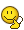I think I got it! Noticing that ##\varepsilon_{imn}\varepsilon^{lmn} B^i B_l = 2\delta_i^l B^i B_l = 2|\vec B_i|^2= -G^{mn}G_{mn}## I get

\begin{align*}
G\cdot G &= \frac 1 4 G_{\mu \nu}G^{\mu \nu} \\
&= \frac 1 4 \left( \underbrace{G_{00}G^{00}}_{=0} + G_{0i}G^{0i} + G_{i0}G^{i0} + G_{jk}G^{jk}\right) \\
&= \frac 1 2 \left( |\vec E_i|^2 -|\vec B_i|^2 \right)
\end{align*}

The -ive sign in front of ##|\vec B_i|^2## still bothers me a bit though...

If you give me the OK I'll proceed to work out ##G\cdot \tilde G##

Homework Helper
Ok, you almost have it. Your problem is that you should be much more careful with raising and lowering indices.

•JD_PM
JD_PM
Ok, you almost have it. Your problem is that you should be much more careful with raising and lowering indices.

I see, we pick up a -ive sign when raising spatial indices so we end up with ##G\cdot G = -\frac 1 2 \left( |\vec E_i|^2 +|\vec B_i|^2 \right)##

Regarding ##G\cdot \tilde G##. I have been thinking for a while on how to work out ##G\cdot \tilde G=\varepsilon_{\mu \nu \rho \sigma} G^{\mu \nu}G^{\rho \sigma}## but I am not quite sure, given that we now have a 4D Levi-Civita symbol so we cannot simply contract it with ##B^i## ...

Homework Helper
It's really not more difficult than what you've just proved. Simply expand the expression as you did, separating temporal and spatial indices and then write ##G## in terms of ##E## and ##B##.
Of course, expanding 4 indices is larger than just 2... But using some logical reasoning and the symmetries of the tensors, you can reduce the amount of work quite a lot.

•JD_PM
JD_PM
\begin{align*}
G \cdot \tilde G &= \varepsilon_{\mu \nu \rho \sigma} G^{\mu \nu} G^{\rho \sigma} \\
&= \varepsilon_{0 i j k} G^{0 i} G^{j k} + \varepsilon_{i 0 j k} G^{i 0} G^{j k} + \varepsilon_{i j 0 k} G^{i j} G^{0 k} + \varepsilon_{i j k 0} G^{i j} G^{k 0} \\
&= 4 \varepsilon_{0 i j k} \varepsilon^{l j k} E^i B_l \\
&= 4 \varepsilon_{i j k} \varepsilon^{l j k} E^i B_l \\
&= 8 E_i \cdot B_i
\end{align*}

The above computation is of course wrong because ##\varepsilon_{0 i j k}## does not equal ##\varepsilon_{i j k}##. I am missing a piece of the puzzle, I should not be working with 3D and 4D LC symbols at once.

Homework Helper
Indeed, what you did is absolutely correct. ##\varepsilon_{0ijk}=\pm\varepsilon_{ijk}##, depending on your definitions. But anyway, your argument is correct.

•JD_PM
JD_PM
Indeed, what you did is absolutely correct. ##\varepsilon_{0ijk}=\pm\varepsilon_{ijk}##, depending on your definitions. But anyway, your argument is correct.
May I ask why that equality holds? I have been reading the wiki page but I found no reference to how LC symbols of different dimensions relate to each other 😕

Homework Helper
The Levi-Civita symbol in 3 dimensions, i.e. ##\varepsilon_{ijk}## is defined by two properties:
• It is completely antisymmetric
• ##\varepsilon_{123}=1##
With those conditions, is easy to see that ##\varepsilon_{ijk}## is completely determined.
Therefore let's define ##\epsilon_{ijk}\equiv \varepsilon_{0ijk}##. Knowing that ##\varepsilon_{\mu\nu\alpha\beta}## have equivalent properties than the 3d one (except that sometimes one defines ##\varepsilon_{0123}=-1##), can you prove that ##\epsilon_{ijk}## is indeed equal to ##\pm\varepsilon_{ijk}##? With the ##\pm## sign if you define ##\varepsilon_{0123}=\pm1##.

•JD_PM
JD_PM
Ahhh so you can simply define ##\epsilon_{ijk} := \varepsilon_{0ijk}##

With the convention ##\varepsilon_{0123} := \pm1## we see that ##\epsilon_{ijk}## indeed satisfied the two LC properties you quoted.

• We note that ##\epsilon_{ijk}## is completely antisymmetric based on the above definition; for instance ##\epsilon_{jik} = \varepsilon_{0jik} = - \varepsilon_{0ijk} = -\epsilon_{ijk}## and so on
• We see that ##\epsilon_{123} = \varepsilon_{0123} := \pm 1##

Hence ##\epsilon_{ijk} = \pm \varepsilon_{ijk}##.

I would be a bit presumptuous if I were to say that the above is a "proof" but at least I understand it now :)

JD_PM
OK so now I understand that the term ##G\cdot \tilde G## breaks CP in QCD. However, the analogous term in QED ( that is ##F\cdot \tilde F##) does not break CP in QED. To understand why, I will read more about it and then post (it seems that it has to do with the fact that the structure of the QCD ground state is more intricate ...).

Homework Helper
OK so now I understand that the term ##G\cdot \tilde G## breaks CP in QCD. However, the analogous term in QED ( that is ##F\cdot \tilde F##) does not break CP in QED. To understand why, I will read more about it and then post (it seems that it has to do with the fact that the structure of the QCD ground state is more intricate ...).
Actually, I'm pretty sure that ##\tilde{F}F## also breaks CP, since both gluons and photons transform the same way under such a transformation. To prove that such a term in the QED Lagrangian is not physically relevant, while in the QCD Lagrangian it affects the physics is a different story...
Anyway, I have no idea how you are supposed to use the ground state here...

JD_PM
Actually, I'm pretty sure that ##\tilde{F}F## also breaks CP, since both gluons and photons transform the same way under such a transformation. To prove that such a term in the QED Lagrangian is not physically relevant, while in the QCD Lagrangian it affects the physics is a different story...

I see. They actually state that ##F \cdot \tilde{F}## term can be dropped as it "amounts to a total divergence".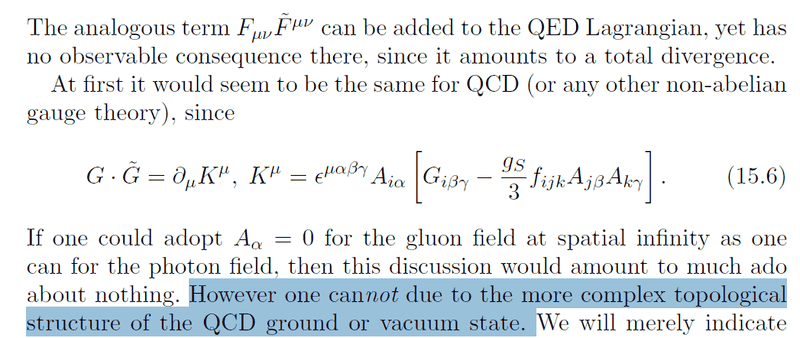I am trying to figure out how to prove that statement before tackling the QCD ground state argument.

•Gaussian97
JD_PM
Alright, I am trying to prove EQ. (15.6) in a purely mathematical way.

Let us recall that repeated pair of indices are summed over and

\begin{equation*}
G_i^{\mu \nu} := F_i^{\mu \nu} + g_s f_{ijk} A_j^{\mu} A_k^{\nu}, \quad F_i^{\mu \nu} := \partial^{\nu} A^{\mu}_i - \partial^{\mu} A^{\nu}_i, \quad G \cdot \tilde G := \varepsilon_{\mu \nu \rho \sigma} G_i^{\mu \nu}G_i^{\rho \sigma}
\end{equation*}

We are allowed to write ##G \cdot \tilde{G} = \partial_\mu K^\mu## because, given the definitions of ##G## and ##\tilde{G}##, it happens to be true that there exists an object ##K^\mu## such that the equality holds. So in order to prove it, we work out ##G \cdot \tilde{G}## explicitly

\begin{align*}
G \cdot \tilde G &= \varepsilon_{\mu \nu \rho \sigma} G_i^{\mu \nu}G_i^{\rho \sigma}\\
&=\varepsilon_{\mu \nu \rho \sigma} (F_i^{\mu \nu} + g_s f_{ijk} A_j^{\mu} A_k^{\nu})(F_i^{\rho \sigma} + g_s f_{ilm} A_l^{\rho} A_m^{\sigma})\\
&=\varepsilon_{\mu \nu \rho \sigma} (F_i^{\mu \nu}F_i^{\rho \sigma} + g_s f_{ilm}F_i^{\mu \nu}A_l^{\rho} A_m^{\sigma} + g_s f_{ijk} A_j^{\mu} A_k^{\nu} F_i^{\rho \sigma} + g^2_sf_{ijk}f_{ilm}A_j^{\mu} A_k^{\nu}A_l^{\rho} A_m^{\sigma})\\
&= ?\\
&= \partial_{\mu}(\varepsilon^{\mu \nu \rho \sigma} A_{\nu}^i [G_{\rho \sigma}^i - \frac{g_s}{3}f_{ijk} A_{j\rho} A_{k\sigma}])
\end{align*}

In order to get to the final result I guess that we will have to make use of the fact that the LC tensor is totally antisymmetric and pull out a derivative ##\partial_{\mu}## but I do not see how...

Any help is appreciatedHomework Helper
Yes, indeed you must use all the symmetries of your tensors. I would suggest you to start by showing that
$$\varepsilon_{\mu\nu\rho\sigma}F_i^{\mu\nu}F^{\rho\sigma}_i = \partial_\mu\left(\varepsilon^{\mu\nu\rho\sigma}A_\nu^i F_{\rho\sigma}^i\right)$$

•JD_PM
JD_PM
Yes, indeed you must use all the symmetries of your tensors. I would suggest you to start by showing that
$$\varepsilon_{\mu\nu\rho\sigma}F_i^{\mu\nu}F^{\rho\sigma}_i = \partial_\mu\left(\varepsilon^{\mu\nu\rho\sigma}A_\nu^i F_{\rho\sigma}^i\right)$$

Mmm I did not quite get it yet.

\begin{align*}
\varepsilon_{\mu\nu\rho\sigma}F_i^{\mu\nu}F^{\rho\sigma}_i &= \varepsilon^{\mu\nu\rho\sigma}F^i_{\mu\nu}F_{\rho\sigma}^i \\
&= \varepsilon^{\mu\nu\rho\sigma}(\partial_{\nu} A_{\mu}^i - \partial_{\mu} A_{\nu}^i)F_{\rho\sigma}^i \\
&= -\varepsilon^{\mu\nu\rho\sigma}\partial_{\mu} A_{\nu}^i F_{\rho\sigma}^i
\end{align*}

Where the first term drops because ##\varepsilon^{\mu\nu\rho\sigma}## is antisymmetric under ##\mu \leftrightarrow \nu## while ##\partial_{\mu} A_{\nu}## is symmetric (not completely sure if this is OK). Next I thought of product rule + divergence theorem

\begin{equation*}
\underbrace{\partial_\mu\left(\varepsilon^{\mu\nu\rho\sigma}A_\nu^i F_{\rho\sigma}^i\right)}_{=0?} =\underbrace{\partial_\mu(\varepsilon^{\mu\nu\rho\sigma})A_\nu^i F_{\rho\sigma}^i}_{=0} + \varepsilon^{\mu\nu\rho\sigma}\partial_\mu A_\nu^i F_{\rho\sigma}^i + \varepsilon^{\mu\nu\rho\sigma}A_\nu^i \partial_\mu F_{\rho\sigma}^i
\end{equation*}

So ##-\varepsilon^{\mu\nu\rho\sigma}\partial_{\mu} A_{\nu}^i F_{\rho\sigma}^i = \varepsilon^{\mu\nu\rho\sigma}A_\nu^i \partial_\mu F_{\rho\sigma}^i## but I do not see how this is going to be helpful...

Homework Helper
Where the first term drops because ##\varepsilon^{\mu\nu\rho\sigma}## is antisymmetric under ##\mu \leftrightarrow \nu## while ##\partial_{\mu} A_{\nu}## is symmetric (not completely sure if this is OK).
Mmm... How would you argue that ##\partial_{\mu}A_{\nu}## is symmetric?? And more important, if the first term vanishes, how in the world the second does not vanish?

Next I thought of product rule + divergence theorem

\begin{equation*}
\underbrace{\partial_\mu\left(\varepsilon^{\mu\nu\rho\sigma}A_\nu^i F_{\rho\sigma}^i\right)}_{=0?} =\underbrace{\partial_\mu(\varepsilon^{\mu\nu\rho\sigma})A_\nu^i F_{\rho\sigma}^i}_{=0} + \varepsilon^{\mu\nu\rho\sigma}\partial_\mu A_\nu^i F_{\rho\sigma}^i + \varepsilon^{\mu\nu\rho\sigma}A_\nu^i \partial_\mu F_{\rho\sigma}^i
\end{equation*}
You shouldn't use the divergence theorem here. First of all, because we are told that the boundary terms don't vanish. And furthermore, we want to express the whole expression as a total divergence, if you use the DT to get rid of such terms...

•JD_PM
JD_PM
And more important, if the first term vanishes, how in the world the second does not vanish?
Absolutely right.

Hence I have

\begin{align*}
\varepsilon_{\mu\nu\rho\sigma}F_i^{\mu\nu}F^{\rho\sigma}_i &= \varepsilon^{\mu\nu\rho\sigma}F^i_{\mu\nu}F_{\rho\sigma}^i \\
&= \varepsilon^{\mu\nu\rho\sigma}(\partial_{\nu} A_{\mu}^i - \partial_{\mu} A_{\nu}^i)F_{\rho\sigma}^i \\
&= -2\varepsilon^{\mu\nu\rho\sigma}\partial_{\mu} A_{\nu}^i F_{\rho\sigma}^i \\
&= -\frac 1 2 \partial_\mu\left(\varepsilon^{\mu\nu\rho\sigma}A_\nu^i F_{\rho\sigma}^i\right) + \frac 1 2 \varepsilon^{\mu\nu\rho\sigma}A_\nu^i \partial_\mu F_{\rho\sigma}^i
\end{align*}

Mmm how to deal with the ##\frac 1 2 \varepsilon^{\mu\nu\rho\sigma}A_\nu^i \partial_\mu F_{\rho\sigma}^i## term though? I thought of Bianchi identity for ##F_{\mu\nu}## but I do not think it is going to lead us to the desired result.

JD_PM
\begin{align*}
G \cdot \tilde G &= \varepsilon_{\mu \nu \rho \sigma} G_i^{\mu \nu}G_i^{\rho \sigma}\\
&=\varepsilon_{\mu \nu \rho \sigma} (F_i^{\mu \nu} + g_s f_{ijk} A_j^{\mu} A_k^{\nu})(F_i^{\rho \sigma} + g_s f_{ilm} A_l^{\rho} A_m^{\sigma})\\
&=\varepsilon_{\mu \nu \rho \sigma} (F_i^{\mu \nu}F_i^{\rho \sigma} + g_s f_{ilm}F_i^{\mu \nu}A_l^{\rho} A_m^{\sigma} + g_s f_{ijk} A_j^{\mu} A_k^{\nu} F_i^{\rho \sigma} + g^2_sf_{ijk}f_{ilm}A_j^{\mu} A_k^{\nu}A_l^{\rho} A_m^{\sigma})\\
&= ?\\
&= \partial_{\mu}(\varepsilon^{\mu \nu \rho \sigma} A_{\nu}^i [G_{\rho \sigma}^i - \frac{g_s}{3}f_{ijk} A_{j\rho} A_{k\sigma}])
\end{align*}

I advanced just a bit: ##A^4## term is symmetric so

\begin{align*}
G \cdot \tilde G &= \varepsilon_{\mu \nu \rho \sigma} G_i^{\mu \nu}G_i^{\rho \sigma}\\
&=\varepsilon_{\mu \nu \rho \sigma} (F_i^{\mu \nu} + g_s f_{ijk} A_j^{\mu} A_k^{\nu})(F_i^{\rho \sigma} + g_s f_{ilm} A_l^{\rho} A_m^{\sigma})\\
&=\varepsilon_{\mu \nu \rho \sigma} (F_i^{\mu \nu}F_i^{\rho \sigma} + g_s f_{ilm}F_i^{\mu \nu}A_l^{\rho} A_m^{\sigma} + g_s f_{ijk} A_j^{\mu} A_k^{\nu} F_i^{\rho \sigma} + g^2_sf_{ijk}f_{ilm}A_j^{\mu} A_k^{\nu}A_l^{\rho} A_m^{\sigma})\\
&=\varepsilon_{\mu \nu \rho \sigma} (F_i^{\mu \nu}F_i^{\rho \sigma} + g_s f_{ilm}F_i^{\mu \nu}A_l^{\rho} A_m^{\sigma} + g_s f_{ijk} A_j^{\mu} A_k^{\nu} F_i^{\rho \sigma})\\
&= ?\\
&= \partial_{\mu}(\varepsilon^{\mu \nu \rho \sigma} A_{\nu}^i [G_{\rho \sigma}^i - \frac{g_s}{3}f_{ijk} A_{j\rho} A_{k\sigma}])
\end{align*}Homework Helper
Absolutely right.

Hence I have

\begin{align*}
\varepsilon_{\mu\nu\rho\sigma}F_i^{\mu\nu}F^{\rho\sigma}_i &= \varepsilon^{\mu\nu\rho\sigma}F^i_{\mu\nu}F_{\rho\sigma}^i \\
&= \varepsilon^{\mu\nu\rho\sigma}(\partial_{\nu} A_{\mu}^i - \partial_{\mu} A_{\nu}^i)F_{\rho\sigma}^i \\
&= -2\varepsilon^{\mu\nu\rho\sigma}\partial_{\mu} A_{\nu}^i F_{\rho\sigma}^i \\
&= -\frac 1 2 \partial_\mu\left(\varepsilon^{\mu\nu\rho\sigma}A_\nu^i F_{\rho\sigma}^i\right) + \frac 1 2 \varepsilon^{\mu\nu\rho\sigma}A_\nu^i \partial_\mu F_{\rho\sigma}^i
\end{align*}

Mmm how to deal with the ##\frac 1 2 \varepsilon^{\mu\nu\rho\sigma}A_\nu^i \partial_\mu F_{\rho\sigma}^i## term though? I thought of Bianchi identity for ##F_{\mu\nu}## but I do not think it is going to lead us to the desired result.
Not sure how you convert the 2 to a 1/2, but the idea is OK. Using the definition of ##F## and symmetry is not difficult to show that
$$\varepsilon^{\mu\nu\rho\sigma}\partial_\mu F_{\rho\sigma}^i=0$$

I advanced just a bit: ##A^4## term is symmetric so
Yes... But I think is not completely trivial to show how the symmetry of ##A^4## cancels this term.

JD_PM
The authors seem to be using the definition ##F_i^{\mu \nu} := \partial^{\mu} A^{\nu}_i - \partial^{\nu} A^{\mu}_i## so let us use it as well from here on.

Not sure how you convert the 2 to a 1/2

Oops indeed, we have

\begin{align*}
&\partial_\mu\left(\varepsilon^{\mu\nu\rho\sigma}A_\nu^i F_{\rho\sigma}^i\right) =\underbrace{\partial_\mu(\varepsilon^{\mu\nu\rho\sigma})A_\nu^i F_{\rho\sigma}^i}_{=0} + \varepsilon^{\mu\nu\rho\sigma}\partial_\mu A_\nu^i F_{\rho\sigma}^i + \varepsilon^{\mu\nu\rho\sigma}A_\nu^i \partial_\mu F_{\rho\sigma}^i \\
&\Rightarrow 2\varepsilon^{\mu\nu\rho\sigma}\partial_\mu A_\nu^i F_{\rho\sigma}^i = 2\partial_\mu\left(\varepsilon^{\mu\nu\rho\sigma}A_\nu^i F_{\rho\sigma}^i\right) - 2\varepsilon^{\mu\nu\rho\sigma}A_\nu^i \partial_\mu F_{\rho\sigma}^i
\end{align*}

Then

\begin{align*}
\varepsilon_{\mu\nu\rho\sigma}F_i^{\mu\nu}F^{\rho\sigma}_i &= \varepsilon^{\mu\nu\rho\sigma}F^i_{\mu\nu}F_{\rho\sigma}^i \\
&= \varepsilon^{\mu\nu\rho\sigma}(\partial_{\mu} A_{\nu}^i - \partial_{\nu} A_{\mu}^i)F_{\rho\sigma}^i \\
&= 2\varepsilon^{\mu\nu\rho\sigma}\partial_{\mu} A_{\nu}^i F_{\rho\sigma}^i \\
&= 2 \partial_\mu\left(\varepsilon^{\mu\nu\rho\sigma}A_\nu^i F_{\rho\sigma}^i\right) - 2 \varepsilon^{\mu\nu\rho\sigma}A_\nu^i \partial_\mu F_{\rho\sigma}^i
\end{align*}

Mmm so there is a 2 factor mismatch between your result and mine.

Using the definition of ##F## and symmetry is not difficult to show that
$$\varepsilon^{\mu\nu\rho\sigma}\partial_\mu F_{\rho\sigma}^i=0$$

\begin{align*}
\varepsilon^{\mu\nu\rho\sigma}\partial_\mu F_{\rho\sigma}^i &= \varepsilon^{\mu\nu\rho\sigma}\partial_\mu(\partial_\rho A_\sigma^i - \partial_\sigma A_\rho^i)\\
&= \varepsilon^{\mu\nu\rho\sigma}\partial_\mu \partial_\rho A_\sigma^i - \varepsilon^{\mu\nu\rho\sigma}\partial_\mu \partial_\sigma A_\rho^i \\
&= 0
\end{align*}

Where we noticed that the object ##\partial_\mu \partial_\rho A_\sigma^i## is symmetric under ##\mu \leftrightarrow \rho## and is contracted with the object ##\varepsilon^{\mu\nu\rho\sigma}##, which is antisymmetric under ##\mu \leftrightarrow \rho##. Hence ##\varepsilon^{\mu\nu\rho\sigma}\partial_\mu \partial_\rho A_\sigma^i = 0##. Analogously, ##\varepsilon^{\mu\nu\rho\sigma}\partial_\mu \partial_\sigma A_\rho^i=0##